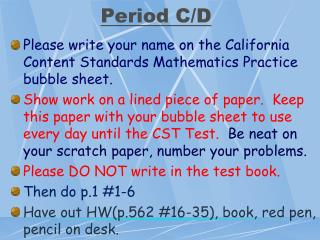DownloadDownload PresentationPeriod C/D

# Period C/D

Download Presentation## Period C/D

- - - - - - - - - - - - - - - - - - - - - - - - - - - E N D - - - - - - - - - - - - - - - - - - - - - - - - - - -
##### Presentation Transcript

1. Period C/D • Please write your name on the California Content Standards Mathematics Practice bubble sheet. • Show work on a lined piece of paper. Keep this paper with your bubble sheet to use every day until the CST Test. Be neat on your scratch paper, number your problems. • Please DO NOT write in the test book. • Then do p.1 #1-6 • Have out HW(p.562 #16-35), book, red pen, pencil on desk.

2. The Pythagorean Theorem p.564 c a • Legs- the twoshortest sides of a right triangle. (a and b) • Hypotenuse- the longest side of a right triangle that is opposite the right angle. (c) b

3. The Pythagorean Theorem c a For any right triangle: b

4. Example Find c, the length of the hypotenuse, in the triangle. c 6 cm 8 cm

5. Example Find c, the length of the hypotenuse, in the triangle. c 6 cm 8 cm

6. Example Find c, the length of the hypotenuse, in the triangle. c 6 cm 36 + 64= c2 8 cm

7. Example Find c, the length of the hypotenuse, in the triangle. c 6 cm 36 + 64= c2 100 = c2 8 cm

8. Example Find c, the length of the hypotenuse, in the triangle. c 6 cm 36 + 64= c2 100 = c2 8 cm

9. Example Find c, the length of the hypotenuse, in the triangle. c 6 cm 36 + 64= c2 100 = c2 8 cm 10 = c

10. Try This Find the length of the missing side of a right triangle. Legs: 3 ft and 4 ft c 3 ft 4 ft

11. Try This Find the length of the missing side of a right triangle. Legs: 3 ft and 4 ft c 5 ft. 3 ft 4 ft

12. Find the length of the missing side. (Hint: is x an a, b, or c?) x 3 in. 5 in.

13. x = b Find the length of the missing side. (Hint: is x an a, b, or c?) x 4 in. 3 in. 5 in.

14. Pythagorean Theorem Review g h i g and h • Which sides of the right triangle are the legs? • Which side of the right triangle is the hypotenuse? • What is always the longest side of any right triangle? • 4) Besides being the longest side, how else do you know that i is the hypotenuse of this right triangle? • 5) What is the formula for the Pythagorean Theorem? i The hypotenuse It is opposite (or across from) the right angle.

15. Agenda • PA #49 • Print out Test Practice from my teacherweb.com • Pp.567-568 • #14, 15, 17-19, 20-28 even

16. Period C/D • Please have the bubble sheet from yesterday and your lined show work paper on your desk. • Be neat on your scratch paper, number your problems. You will turn in this paper for class points. • Do p.2 #7-14 • DO NOT write in the test book. • Have out HW (p.567), book, red pen, pencil on desk.

17. Agenda • PA #50 • Print out Test Practice from my teacherweb.com & do #1-10 • Workbook pp.92 evens and 93 evens

18. Period C/D • Please have out your bubble sheet and lined show work paper on your desk. • Be neat on your scratch paper, number your problems. You will turn in this paper for class points. • Do p.3 #15-22 • DO NOT write in the test book. • Have out HW (test practice and workbook) red pen, pencil on desk.

19. Agenda • PA #51 • Worksheet 13-4 #1-16 • Worksheet 12-2 #1-6 Using the Pythagorean Theorem • Quiz tomorrow.

20. Period C/D • Please have out your bubble sheet and lined show work paper on your desk. • Be neat on your scratch paper, number your problems. You will turn in this paper for class points. • Do p.4 #23-27 • DO NOT write in the test book. • Have out HW (worksheets) red pen, pencil on desk.

21. Agenda • PA #52 • Print out Test Practice from my teacherweb.com & do #11-20 • Worksheet 3-6